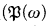Home

# Maximal irredundance and maximal ideal independence in Boolean algebras

## Extract

Recall that a subset X of an algebra A is irredundant iff x ∉ 〈X∖{x}〉 for all x ϵ X, where 〈X∖{x}) is the subalgebra generated by X∖{x}. By Zorn's lemma there is always a maximal irredundant set in an algebra. This gives rise to a natural cardinal function Irrmm(A) = min{∣X∣: X is a maximal irredundant subset of A}. The first half of this article is devoted to proving that there is an atomless Boolean algebra A of size 2ω for which Irrmm(A) = ω.

A subset X of a BA A is ideal independent iff x ∉ (X∖{x}〉id for all x ϵ X, where 〈X∖{x}〉id is the ideal generated by X∖{x}. Again, by Zorn's lemma there is always a maximal ideal independent subset of any Boolean algebra. We then consider two associated functions. A spectrum function

Sspect(A) = {∣X∣: X is a maximal ideal independent subset of A}

and the least element of this set, smm(A). We show that many sets of infinite cardinals can appear as Sspect(A). The relationship of Smm to similar “continuum cardinals” is investigated. It is shown that it is relatively consistent that Smm/fin) < 2ω.

We use the letter s here because of the relationship of ideal independence with the well-known cardinal invariant spread; see Monk . Namely, sup{∣X∣: X is ideal independent in A} is the same as the spread of the Stone space Ult(A); the spread of a topological space X is the supremum of cardinalities of discrete subspaces.

Hide All

# Maximal irredundance and maximal ideal independence in Boolean algebras

## Metrics

### Full text viewsFull text views reflects the number of PDF downloads, PDFs sent to Google Drive, Dropbox and Kindle and HTML full text views.

Total number of HTML views: 0
Total number of PDF views: 0 *Loading metrics...

### Abstract viewsAbstract views reflect the number of visits to the article landing page.

Total abstract views: 0 *Loading metrics...

* Views captured on Cambridge Core between <date>. This data will be updated every 24 hours.

Usage data cannot currently be displayed.